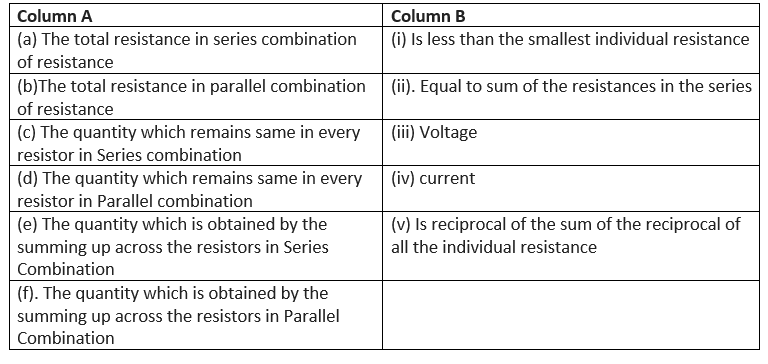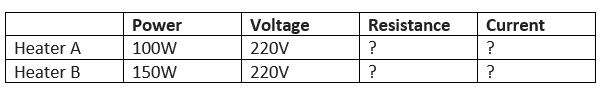# Class 10 Science|physics Important Questions for Electricity

Given below are the Class 10 Science Important Questions for electricity
(a) Very Short Answer Based questions
(b) Match the column
(c) Table type question

## Very Short Answer Based questions

1. What is relation between KWH and Joule?
2. The Flow of electron constitutes the electric current in the wire.  True or false?
3. A voltmeter has high resistance and it is connected in parallel  True of false?
4. What is resistance of the conductor?
5. What is the SI unit of resistivity?
6. What are the applications of chemical effect of current?
7. What is same across the parallel combination of resistors Voltage or current?
8. What is critical temperature in metals?

## Match the column## Table type question

Two heaters A and B are givenSome more questions to be answered based on above data
1. Which Heater for high resistance
2. If 1KWH is priced at 30 paise,which heater  will be turned costlier if they run for 1 hours each

Question 1.
What happens to resistance when length of conductor is doubled without affecting the thickness of conductor?

Question 2.
What is the lowest resistance that can be obtained by combining four coils of resistors of 4 Ω, 8 Ω, 12 Ω and 24 Ω?

Question 3.
What is an ammeter?
Question 4.
Calculate the number of electrons constituting one coulomb of charge.

Question 5.
A voltmeter is to be connected in the circuit to measure potential difference across a conductor. Mention the type of combination in which it should be conducted with the conductor.
Question 6.
A bulb cannot be used in place of a resistor to verify Ohm’s law. Justify this statement with reason.
Question 7.
What is meant by electric resistance of a conductor?
Question 8.
Define resistance of a conductor.
Question 9.
Write SI unit of resistivity.
Question 10.
How does the resistivity of alloys compare with those of pure metals form which they may have formed?
Question 11.
Mention any two factors on which the resistance of a cylindrical conductor depends.
Question 12.
Write the relation between resistance R of filament of a bulb, its power (P) and a constant voltage V applied across it.

Question 13.
Power of a lamp is 100 W. Find the energy in joules consumed by it in 1 s.

Question 14.
When a 12 V battery is connected across an unknown resistor, 2.5 mA current flows in the circuit. Find the resistance of the resistor.

Question 15.
State the factors on which the resistance of a cylindrical conductor depends. Hence, define resistivity.
Question 16.
In an electric circuit with a resistance wire and a cell, the current flowing is I. What would happen to this current if the wire is replaced by another thicker wire of same material and same length. Give reason.

Question 17.
A wire of length L and resistance R is stretched so that the length is doubled and area of cross – section halved. How will
(i) resistance change, and
(ii) resistivity change.

Question 18.
(a) What material is used in making the filament of an electric bulb?
(b) Name the characteristics which make it suitable for this.
Question 19.
An electric lamp is marked 220 V, 100W. It is used for 5 hours daily. Calculate:
(a) Its resistance while glowing.
(b) Energy consumed in kW h/day.

Question 20.
List in a tabular form two differences between a voltmeter and an ammeter.
Question 21.
A thick wire and a thin wire the same material are successively connected to the same circuit to find their respective resistance. Which one will have lower resistance? Give reason.
Question 22.
State the factors on which the heat produced in a current carrying conductor depends. Give one practical application of this effect.
Question 23.
Write the relation between electric power (W) of a device with potential difference (Volt) across it and current (I) flowing through it.

Question 24.
What is electrical resistivity? In a series circuit comprising of a resistor having a metallic wire, the ammeter reads 5 A. The reading of the ammeter decrease to half when the length of the wire is doubled. Why?

Question 1.
Draw the symbols of commonly used components in electric circuit diagrams for:
(i) An electric cell
(ii) open plug key
(iii) Wires crossing without connection or joining
(iv) Variable resistor
(v) Battery
(vi) Electric bulb
(vii) Resistance
(viii) Wire joint

Question 2.
Draw a labeled circuit diagram to study a relationship between the current (I) flowing through a conductor and the potential difference (V) applied across its two ends. State the formula correlating the I in a conductor and the V across it. also show this relationship by drawing a diagram.
Question 3.
In electric potential difference a scalar or vector quantity? What do you mean by a potential difference of 1 volt?

Question 4.
a. What does an electric circuit mean?
b. Name a device that helps to maintain a potential difference across a conductor in a circuit.
c. When do we say that the potential difference across a conductor is 1 volt?
d.Calculate the amount of work done in shifting a charge of 2 coulombs from a point A to B having potentials 10 V and 5V respectively.

Question 5.
State Ohm's law. How can it be verified experimentally? Does it hold good in all conditions? Comment.
Question 6.
Express Joule's law of heating mathematically.
Question 7.
What is the resistance of 12 m wire having radius 2×10-4 m and resistivity 3.14×10-8 Ω-m.

Question 6.
A 4Ω resistance wire is doubled on it. Calculate the new resistance of the wire.

Question 7.
a.Define 1 volt. Express it in terms of SI unit of work and charge.
b. Calculate the amount of energy consumed in carrying a charge of 1 coulomb through a battery of 3 volts.

Question 8.
The resistance per meter length of a wire is 10 Ω. If the resistivity of the material of the wire is 50×10-8 ohm meters, find the area of cross – section of the wire.

Question 9.
When a resistor R is connected to a battery of 3 V, it draws a current of 1 ampere. find the value of R. If an identical resistor is connected in parallel with it, find the current that flows through the circuit?.

Question 10.
Derive an expression for the equivalent resistance of three resistors R1, R2 and R3 connected in parallel.

Question 11.
Christmas tree lamps are usually connected in series. What will be if one lamp breaks?

Question 12.
With the help of a neat diagram derive the expression for the effective resistance when three resistors R1, R2 and R3 are connected is series.

Question 13.
What would be the resistance of a resistor if the current flowing through it is 0.15 A when the potential difference across it is 1.05 V?

Question 14.
(a) Name and state the law that gives relationship between the potential difference (V) across the two ends of a conductor and the current (I) flowing through it.
(b) Represent it (Ohm's law) mathematically.
(c) Draw a circuit diagram for the verification of Ohm's law.
(d) Draw the V - I graph for this (Ohm's) law.

Question 15.
State and explain Ohm's law. Define resistance and give its SI unit. What is meant by 1 ohm resistance? Draw V - I graph for an ohmic conductor and list its two important features.

Question 16.
What is meant by resistance of a conductor? Name and define its SI unit. List the factors on which the resistance of a conductor depends. How is the resistance of a wire affected if:
(i) its length is doubled

Question 17.
List two distinguishing features between the resistance and resistivity of a conductor. A wire is stretched so that its length becomes 6/5 times of its original length. If its original resistance is 25 Ω find its new resistance. Give justification for your answer in each case.